The inverse of 3 x 3 matrices with matrix row operations

The inverse of 3 x 3 matrices with matrix row operations

In this section, you will learn how to find the inverse of a 3 x 3 matrix. This method requires the use of matrix row operation. The idea is to draw a vertical line in the middle, write the matrix on the left side of the line, and write the 3 x 3 identity matrix on the right side of the line. Then the goal is to do a bunch of matrix row operation so that the identity matrix appears on the left side of the line. Whatever appears on the right side will be the inverse. Note that this method works for 2 x 2 matrices as well, except the right side of the line is a 2 x 2 identity matrix.

Lessons

This is a lot different from finding inverses of 2 x 2 matrices from the previous section, so start with a fresh mind when finding the inverse of 3 x 3 matrices. This method also works for any square matrix!
• Introduction
The Inverse of 3 x 3 Matrix using matrix row operations

• 1.
Finding the Inverse
You are given that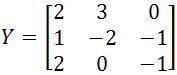. Find the inverse of this matrix.

• 2.
You are given that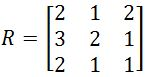. Find the inverse of this matrix.

• 3.
You are given that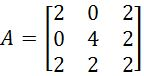. Find the inverse of this matrix.

• 4.
You are given that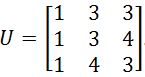. Find the inverse of this matrix.

• 5.
You are given that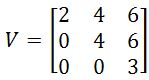. Find the inverse of this matrix.